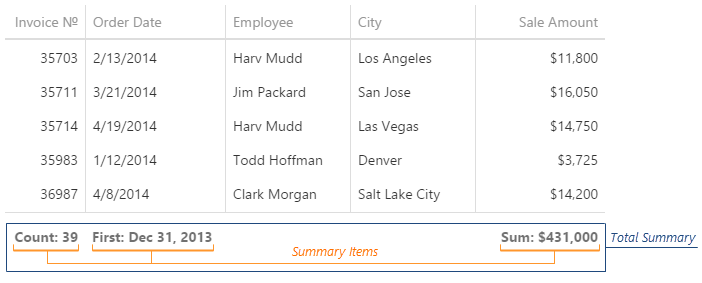# Total Summary

A total summary aggregates all data by columns. You can associate each column with one or more summary items of different types.Configure each summary item in the summary.totalItems array. The basic item configuration requires specifying a column that provides data and the aggregate function. Note that each summary item is calculated only for those rows that meet filtering conditions (if a filter is applied).

##### jQuery
JavaScript
```\$(function () {
\$("#dataGridContainer").dxDataGrid({
// ...
columns: ["OrderNumber", "Price"],
summary: {
totalItems: [{
column: "OrderNumber",
summaryType: "count"
}, {
column: "Price",
summaryType: "sum"
}
// ...
]
}
});
});```
##### Angular
HTML
TypeScript
```<dx-data-grid ...
[columns]="['OrderNumber', 'Price']" >
<dxo-summary>
<dxi-total-item
column="OrderNumber"
summaryType="count">
</dxi-total-item>
<dxi-total-item
column="Price"
summaryType="sum">
</dxi-total-item>
</dxo-summary>
</dx-data-grid>```
```import { DxDataGridModule } from "devextreme-angular";
// ...
export class AppComponent {
// ...
}
@NgModule({
imports: [
// ...
DxDataGridModule
],
// ...
})```

## Alignment and Location

A summary item is under the column providing data and has the same alignment as this column's data cells by default. Use the showInColumn and alignment options to change the default values.

##### jQuery
JavaScript
```\$(function () {
\$("#dataGridContainer").dxDataGrid({
// ...
summary: {
totalItems: [{
column: "Amount",
summaryType: "avg",
showInColumn: "StoreCity",
alignment: "center"     // or "left" | "right"
},
// ...
]
}
});
});```
##### Angular
HTML
TypeScript
```<dx-data-grid ... >
<dxo-summary>
<dxi-total-item
column="Amount"
summaryType="avg"
showInColumn="StoreCity"
alignment="center">     <!-- or "left" | "right" -->
</dxi-total-item>
</dxo-summary>
</dx-data-grid>```
```import { DxDataGridModule } from "devextreme-angular";
// ...
export class AppComponent {
// ...
}
@NgModule({
imports: [
// ...
DxDataGridModule
],
// ...
})```